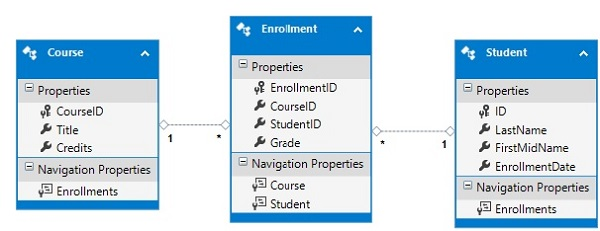# Entity Framework - Database Setup

In this tutorial, we will be using a simple University database. A University database can be much more complex as a whole but for demo and learning purpose, we are using the simplest form of this database. The following diagram contains three tables.

• Student
• Course
• EnrollmentWhenever a term database is used one thing comes directly to our mind and that is different kind of tables which has some sort of relationship. There are three types of relationships between tables and the relationship between different tables depends on how the related columns are defined.

• One-to-Many Relationship
• Many-to-Many Relationship
• One-to-One Relationship

## One-to-Many Relationship

One-to-many relationship is the most common type of relationship. In this type of relationship, a row in table A can have many matching rows in table B, but a row in table B can have only one matching row in table A. For example, in the above diagram, Student and Enrollment table have one-to-many relationship, each student may have many enrollments, but each enrollment belongs to only one student.

## Many-to-Many Relationship

In a many-to-many relationship, a row in table A can have many matching rows in table B, and vice versa. You create such a relationship by defining a third table, called a junction table, whose primary key consists of the foreign keys from both table A and table B. For example, Student and Course table have many-to-many relationship that is defined by a one-to-many relationship from each of these tables to the Enrollment table.

## One-to-One Relationship

In one-to-one relationship, a row in table A can have no more than one matching row in table B, and vice versa. A one-to-one relationship is created if both of the related columns are primary keys or have unique constraints.

This type of relationship is not common because most information related in this way would be all-in-one table. You might use a one-to-one relationship to −

• Divide a table with many columns.
• Isolate part of a table for security reasons.
• Store data that is short-lived and could be easily deleted by simply deleting the table.
• Store information that applies only to a subset of the main table.